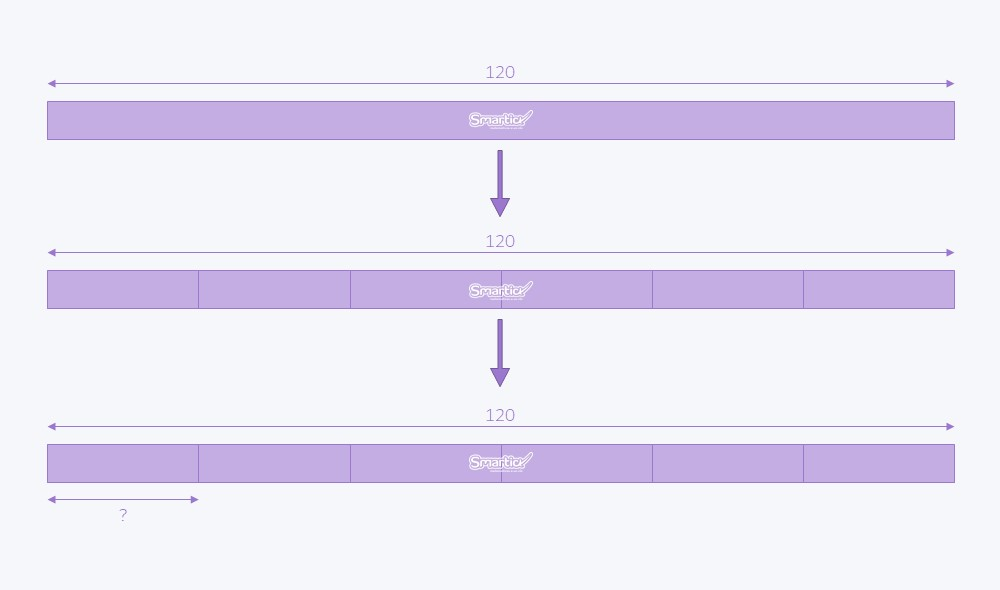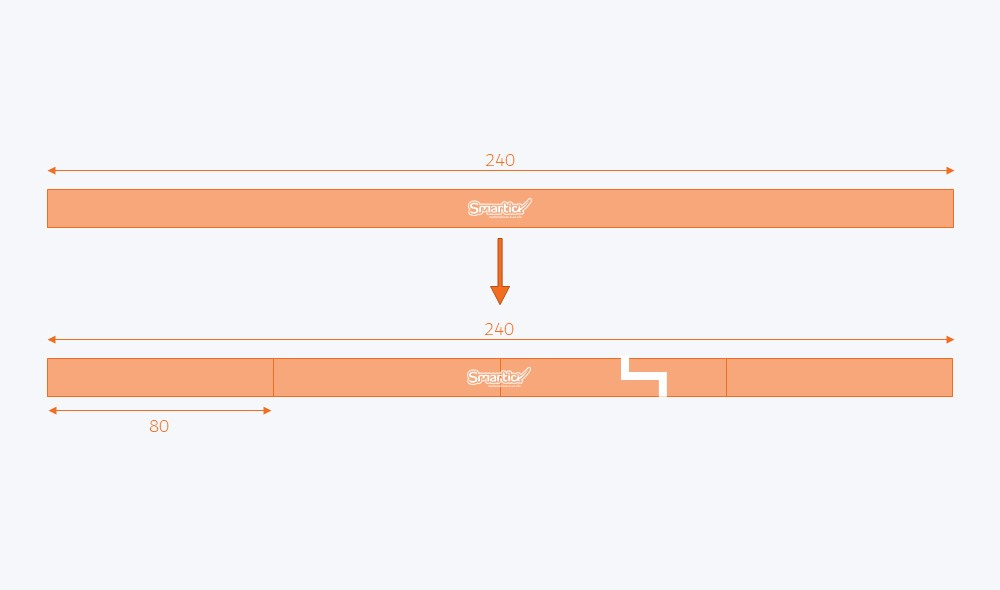Smartick is a fun way to learn math!May14

# Division Exercises Solved Using the Singapore Method

In this post, we’re going a reference a previous one where we looked at the different types of division problems that exist according to how the problem is formulated. Taking these problems as examples, we’re going to look at different strategies to solve division exercises using the Singapore method.

#### The first are division exercises involving repetition or equal groups:

There are 120 books on the 6 shelves of the bookshelf in my living room. Knowing that each shelf has the same number of books on it, calculate how many books there are on each shelf.We represent the total number of objects, the 120 books, with a bar divided into 6 equal parts, one for each shelf.

We want to know how many books there are on each shelf, which is why one of the sections of the bar is labeled with a question mark.

We need to divide 120 by 6, which is 20. There are 20 books on each shelf.

#### The following type of division exercises are the results of problems involving scales of comparison or multiplicative comparison:

The bus from Chicago to Luis's town costs 12 dollars, which is exactly 3 times more than it costs to go from Chicago to Marta's town. How much does it cost to get the bus from Chicago to Marta's town?First, we represent the price of the bus ticket to Luis’s town with a bar.

Then we represent the cost of the ticket to Marta’s town with a bar that is three times smaller than the first one.

We need to divide 12 by 3, which is 4. The bus to Marta’s town costs 4 dollars.

#### The last type of division exercises we’re going to look at are the result of problems involving scales of formulas or reasoning:

Paul is a train driver. He told me that he drives 240 miles on each journey along his line and that he travels at a speed of 80 miles per hour. How long does it take him to travel from one end of the line to the other?We need a somewhat deeper understanding of the method in order to model these types of division exercises.

We start the same way as in the previous exercises, drawing a bar that represents the miles traveled each day.

When dividing the bar into units, the number of parts we make will be inexact because that’s what we’re trying to work out, which is why we broke the bar.

The total is 240, which is divided into sections of 80, but we don’t know how many.

To calculate it, we need to divide 240 by 80, which is 3. In other words, it takes 3 hours to travel from one end of the line to the other.

I hope you’ve found this post interesting and that it’s helped you learn how to model division problems. That way it’s much easier to solve them!